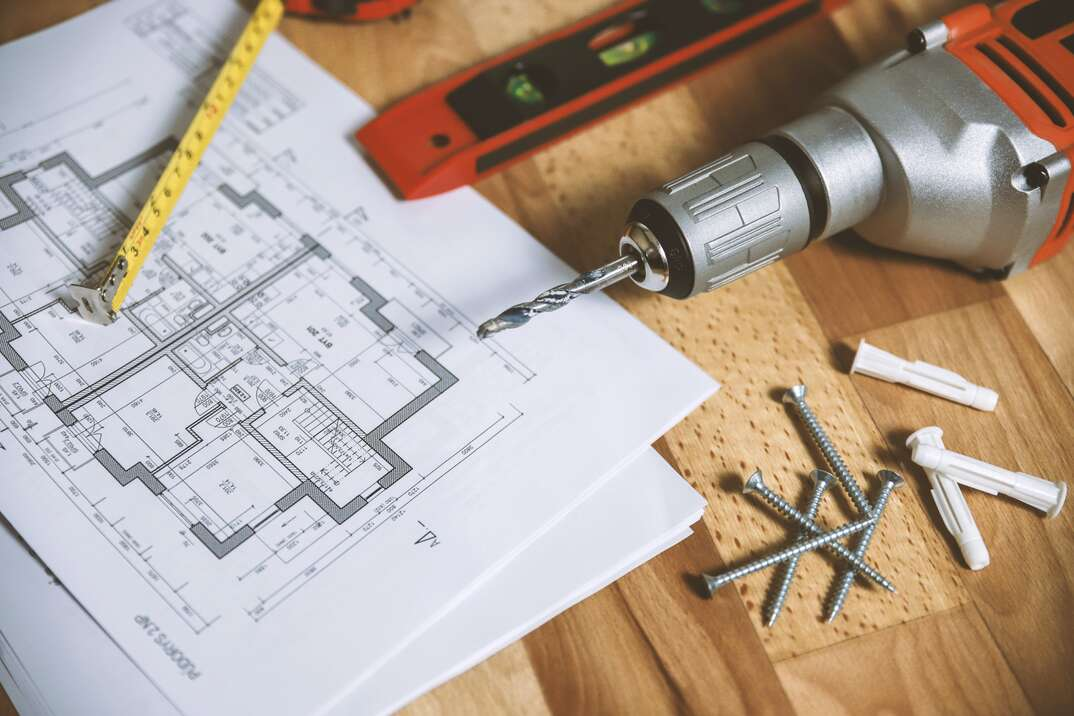# How to Calculate Square Footage

by Team HomeServePainting a room? Laying flooring? Installing a new driveway? Replacing your roof? These are just some of the instances where you’ll need to calculate square footage. Many contractors charge per square foot for labor. Materials like flooring, tile, sod, drywall and siding are often sold by the square foot.

This May Also Interest You: Carpet Installation and Replacement Cost

The list of places you’ll find this measurement used is endless. Knowing the square footage of an area can help you better estimate project cost. Here’s how to calculate the square footage of your space.

## What Is a Square Foot?

A square foot is a measurement of area. It’s defined as the area inside a square that measures one foot per side. Area is equal to length multiplied by width. So, one square foot is equal to an area that's one foot long by one foot wide.

## How Do You Calculate Square Footage?

All home projects — from wallpapering to landscaping — start with proper measurements. To find the square footage of a space, you'll need a measuring tape and a method of recording your measurements. Here are the steps:

1. Start with the longest side of the room or space and measure the length. Be sure your tape measure is in a straight line.
2. Write down the length in feet.
3. Repeat the process on the shortest side of the room or space you are measuring. For square spaces, all four sides will be the same.
4. Write down the width in feet.
5. Multiply the two values together to get square feet.

## How to Calculate Square Footage of Spaces of Different Shapes

The square footage formula depends on the shape of the space. Here are the formulas for some common shapes:

### Rectangular and Square Spaces

Formula: Area = l x w

Plug your measurements in for length and width and calculate as described above.

### Circular Spaces

Formula: Area = πr2

Measure the radius, which is the distance from the midpoint of the space to the edge. The radius is half the diameter — or the length of a straight line that passes through the center of the circle.

Next, square the radius and multiply it by pi (π), or 3.14.

### Triangular Spaces

Formula: Area = ½bh

This formula works with right triangles, which have one 90-degree angle. If your space isn’t already a right triangle, break it into two right triangles for the purposes of measuring.

For each right triangle, multiply the lengths of the straight sides — the base and height. Then, divide by two. With multiple triangles, add the values for the total square footage.

More Related Articles:

## How to Calculate Square Footage of Spaces With Odd Dimensions

Unfortunately, some spaces aren’t a neat geometric shape. You can divide the space into smaller shapes to get an accurate measure of square footage. Then, add all the calculations to get the total area.

For example, if you have a rectangular kitchen with a breakfast nook, you can measure the main part of the room and the nook separately, then add the results to get the total square footage.

First, find the length and width of each section:

• Main room: 12 feet x 10 feet = 120 square feet
• Nook: 4 feet x 6 feet = 24 square feet

120 square feet + 24 square feet = 144 square feet

## Calculating Square Footage of Materials: How Much Should You Adjust for Waste?

After you cut tiles, wallpaper or other materials you may have odd pieces left over that you cannot use. You need to order enough material to account for some waste to ensure you have enough to finish the project. Also, it makes sense to pad your estimate to cover any mistakes that occur.

A general rule of thumb is to order 5% to 10% more material than the square footage of your space. If you end up with a lot of extra material at the end of your project, you can keep some in case repairs are needed down the road. Most stores will also accept returns of unopened material.

## How Do You Calculate Square Footage of a Whole House?

Square footage of a home includes finished, livable areas. In calculating the square footage of your home, you typically don’t include an unfinished basement, attic or garage. Outbuildings are also left out of this measurement. You should, however, include hallways, stairwells and closets in the square footage.

Measure each room or space individually like this:

• Bedroom 1: 10 feet x 12 feet = 120 square feet
• Bedroom 2: 10 feet x 12 feet = 120 square feet
• Living room: 12 feet x 15 feet = 180 square feet
• Bathroom: 5 feet x 7 feet = 35 square feet
• Kitchen: 10 feet x 12 feet = 120 square feet
• Dining room: 10 feet by 10 ft = 100 square feet
• Front hall: 4 feet by 10 feet = 40 square feet
• Closet: 1 foot by 5 feet = 5 square feet

Then, add them together for total square footage. In this case, this house is 720 square feet.

## Convert Inches to Feet

You may need to convert inches to feet for certain projects. To do so, divide the total number of inches by 12. To get from feet to inches, multiply by 12.

Since we’re all home now more than ever, being prepared for unexpected home repairs with a plan from HomeServe is important. Having a plan in place gives you the peace of mind knowing that you can simply call our 24/7 repair hotline for covered breakdowns. See what plans are available in your neighborhood.